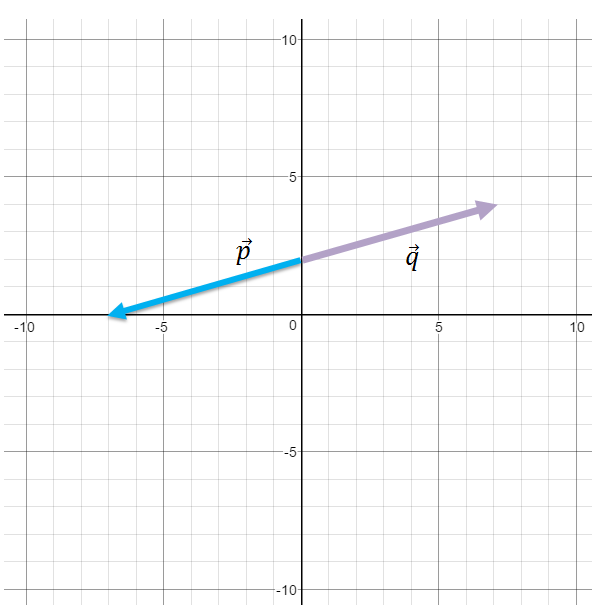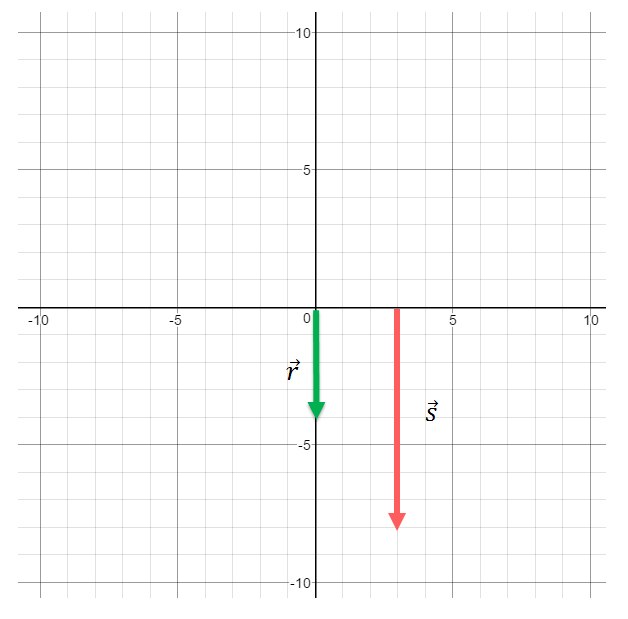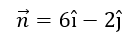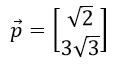# Magnitude of a vector

### Magnitude of a vector

Finding the magnitude of a vector is very much the same as determining the length of the hypotenuse of a right triangle – we almost use the exact Pythagorean formula! In this section, we will learn how the horizontal component and the vertical component enable us to algebraically calculate the magnitude of a vector.

#### Lessons

Magnitude of a vector
$|| \vec{v}||=\sqrt{v^2_x+v^2_y}$
• 1.
Find the magnitude of the following vectors:a)
$\vec{p}$

b)
$\vec{q}$

• 2.
Find the magnitude of the following vectors:a)
$\vec{r}$

b)
$\vec{s}$

• 3.
Find the magnitude of the following vectors:
a)
$\vec{m}=$< $3,-1$>

b)c)• 4.
Given that the vector $\vec{v}$ has its initial point at (2,1) and its terminal point at (6,-4), find the magnitude of the vector $\vec{v}$